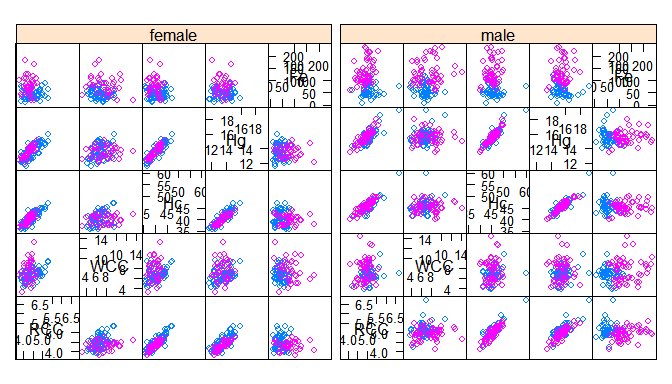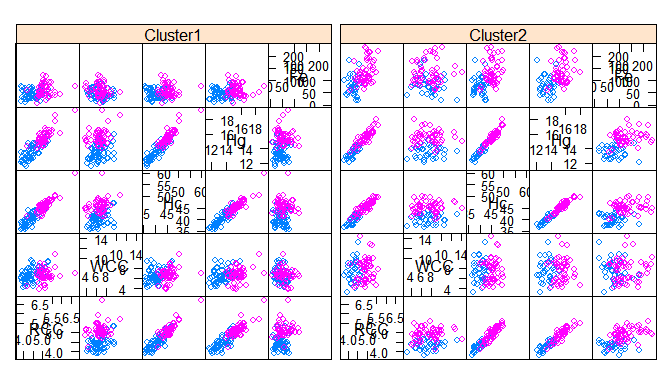# MoEClust: Finite Gaussian Mixtures of Experts - Parsimonious Model-Based Clustering with Gating and Expert Network Covariates

## Introduction

MoEClust is an R package which fits finite Gaussian Mixtures of Experts models with mclust family covariance structures using the EM algorithm, ie. allows incorporation of covariates into the mixing proportions and/or Gaussian densities of finite mixture models under the various parsimonious covariance parameterisations in the mclust family. These models were introduced by Murphy and Murphy (2017). The package also visualises mixture of experts models with parsimonious covariance parameterisations using generalised pairs plots.

The most important function in the MoEClust package is: MoE_clust, for fitting the model via the EM algoirthm with gating and/or expert network covariates, supplied via formula interfaces. Other functions also exist, e.g. MoE_control, MoE_crit, MoE_dens, MoE_estep, and MoE_aitken, which are all used within MoE_clust but are nonetheless made available for standalone use. MoE_compare is provided for conducting model selection between different results from MoE_clust using different covariate combinations &/or initialisation strategies, etc.

A dedicated plotting function exists for visualising the results using generalised pairs plots, for examining the gating network &/or log-likelihood, and/or graphing model selection criteria values. The generalised pairs plots (MoE_gpairs) visualise all pairwise relationships between clustered response variables and associated gating &/or expert network continuous &/or categorical variables, coloured according to the MAP classification, and also give the marginal distributions of each variable along the diagonal.

An as.Mclust method is provided to coerce the output of class "MoEClust" from MoE_clust to the "Mclust" class, to facilitate use of plotting and other functions for the "Mclust" class within the mclust package. As per mclust, MoEClust also facilitates modelling with an additional noise component (with or without the mixing proportion for the noise component depending on covariates).

The package also contains two data sets: ais and CO2data.

If you find bugs or want to suggest new features please visit the MoEClust GitHub issues page. This vignette aims to demonstrate the MoEClust models via application to well-known univariate and multivariate data sets provided with the package.

### Installing MoEClust

MoEClust will run in Windows, Mac OS X or Linux. To install it you first need to install R. Installing Rstudio as a nice desktop environment for using R is also recommended.

Once in R you can type at the R command prompt:

install.packages('devtools')
devtools::install_github('Keefe-Murphy/MoEClust')

to install the latest development version of the package from the MoEClust GitHub page.

To instead install the latest stable official release of the package from CRAN go to R and type:

install.packages('MoEClust')

In either case, if you then type:

library(MoEClust)

it will load in all the MoEClust functions.

The GitHub version contains a few more features but some of these may not yet be fully tested, and occasionally this version might be liable to break when it is in the process of being updated.

## CO2 Data

data(CO2data)
GNP   <- CO2data[,1]
CO2   <- CO2data[,2]

Fit various mixture models to cluster the CO2 data, allowing the GNP variable enter the gating &/or expert networks, or neither. Note that for models with covariates in the gating network, or models with equal mixing proportions, we don’t need to fit single-component models (though it could be done!) as this would merely duplicate the single-component models within m1 and m3, respectively. For the model with no covariates, we can only fit a model with only a noise component by including G=0.

m1    <- MoE_clust(CO2, G=0:2, verbose=FALSE)
m2    <- MoE_clust(CO2, G=2,   gating= ~ GNP, verbose=FALSE)
m3    <- MoE_clust(CO2, G=1:2, expert= ~ GNP, verbose=FALSE)
m4    <- MoE_clust(CO2, G=2,   gating= ~ GNP, expert= ~ GNP, verbose=FALSE)
m5    <- MoE_clust(CO2, G=2,   equalPro=TRUE, verbose=FALSE)
m6    <- MoE_clust(CO2, G=2,   expert= ~ GNP, equalPro=TRUE, verbose=FALSE)

Choose the best model among these and examine the results.

(comp <- MoE_compare(m1, m2, m3, m4, m5, m6, pick=5))
## ------------------------------------------------------------------------------
## Comparison of Gaussian finite mixture of experts models fitted by EM algorithm
## Data: CO2
## ------------------------------------------------------------------------------
##
##  rank MoENames modelNames G df     bic     icl     aic gating expert equalPro
##     1       m3          V 2  7  -157.2 -160.04 -147.88   None   ~GNP    FALSE
##     2       m3          E 2  6 -158.84  -162.5 -150.85   None   ~GNP    FALSE
##     3       m4          V 2  8 -159.25 -161.47 -148.59   ~GNP   ~GNP    FALSE
##     4       m4          E 2  7  -160.2 -163.76 -150.88   ~GNP   ~GNP    FALSE
##     5       m6          E 2  5 -160.27 -164.49 -153.61   None   ~GNP     TRUE

(best <- comp$optimal) ## Call: MoE_clust(data = CO2, G = 1:2, expert = ~GNP, verbose = FALSE) ## ## Best Model: univariate, unequal variance (V), with 2 components ## BIC = -157.2 | ICL = -160.04 | AIC = -147.88 ## Including expert network covariates: ## Expert: ~GNP (summ <- summary(best)) ## --------------------------------------------------------------- ## Gaussian finite mixture of experts model fitted by EM algorithm ## Data: CO2 ## --------------------------------------------------------------- ## ## MoEClust V (univariate, unequal variance), with 2 components ## ## Equal Mixing Proportions: FALSE ## Noise Component: FALSE ## Gating Network Covariates: None ## Expert Network Covariates: ~GNP ## ## log.likelihood n d df BIC ICL AIC ## -66.94 28 1 7 -157.2 -160.04 -147.88 ## ## Clustering table: ## 1 2 ## 6 22 Visualise the results for the optimal model using a generalised pairs plot. plot(comp$optimal, what="gpairs", jitter=FALSE)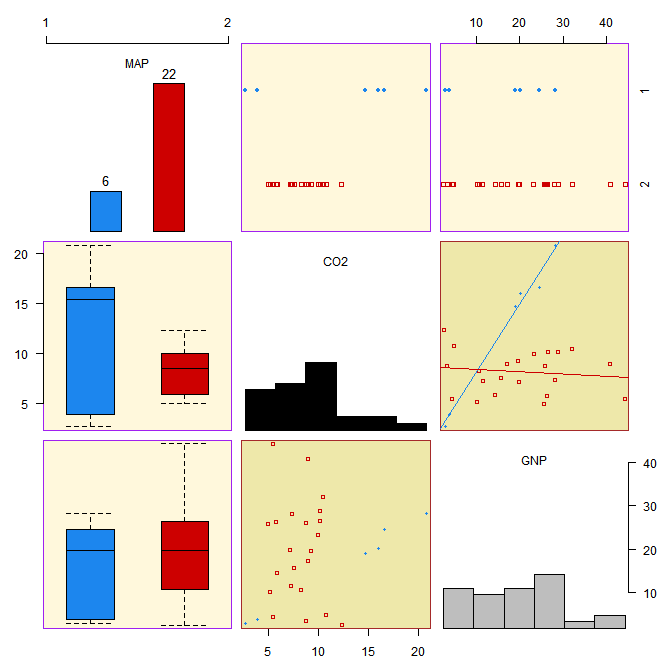Visualise the density of the mixture distribution.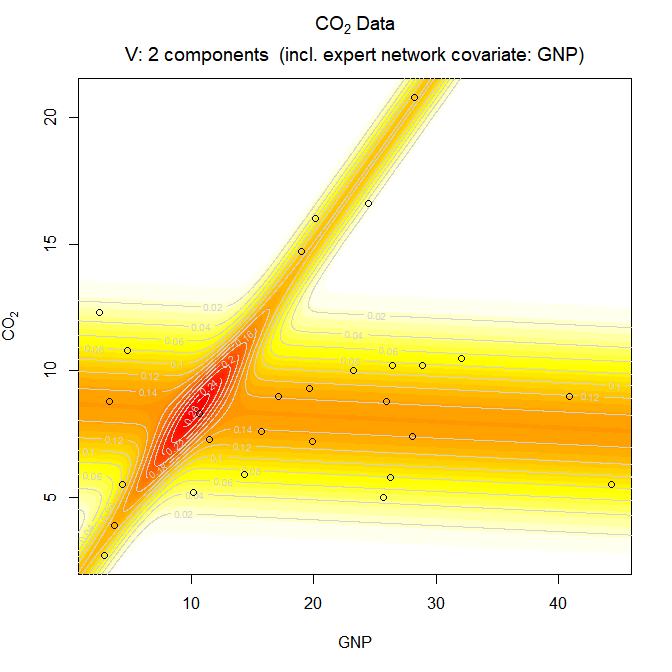Convert from the "MoEClust" class to the "Mclust" class in order to further visualise the results. Examine the "classification" and "uncertainty" options.

(mod <- as.Mclust(comp$optimal)) ## 'Mclust' model object: (V,2) ## List of 15 ##$ call : language MoE_clust(data = CO2, G = 1:2, expert = ~GNP, verbose = FALSE)
## $data : num [1:28, 1] 14.7 3.9 20.8 9 8.3 16 7.6 7.4 10.2 10.8 ... ##$ modelName : chr "V"
## $n : int 28 ##$ d : int 1
## $G : int 2 ##$ BIC : mclustBIC [1:2, 1:2] -166 -159 NA -157
## $bic : num -157 ##$ loglik : num -66.9
## $df : atomic [1:1] 7 ##$ hypvol : logi NA
## $parameters :List of 4 ##$ z : num [1:28, 1:2] 9.91e-01 9.19e-01 1.00 7.50e-135 4.35e-01 ...
## $classification: num [1:28] 1 1 1 2 2 1 2 2 2 2 ... ##$ uncertainty : num [1:28] 9.49e-03 8.12e-02 4.71e-09 0.00 4.35e-01 ...
plot(mod, what="classification")
plot(mod, what="uncertainty")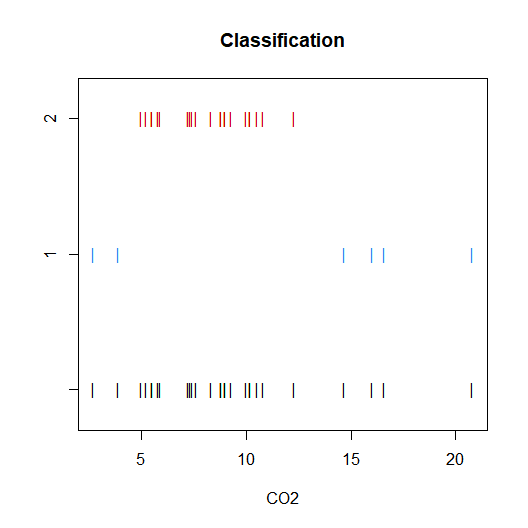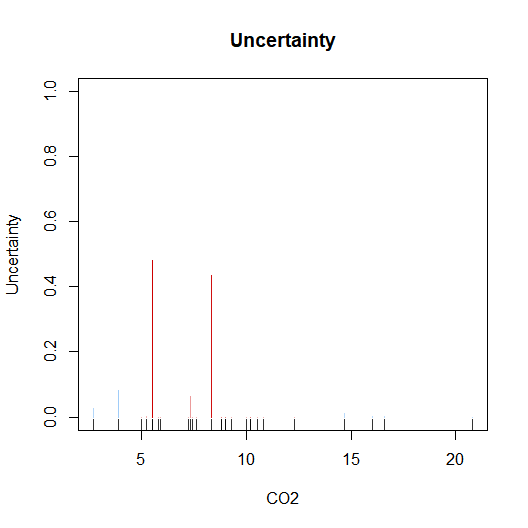## AIS Data

Load the Australian Institute of Sports data.

data(ais)
hema  <- ais[,3:7]
sex   <- ais$sex bmi <- ais$BMI

Fit a mixture of experts model to the hematological variables within AIS data, with sex in the expert network and bmi in the gating network. This time, allow the printing of messages to the screen.

mod   <- MoE_clust(hema, G=1:3, expert= ~ sex, gating = ~ bmi)

Visualise the results for the optimal model using a generalised pairs plot.

plot(mod, what="gpairs")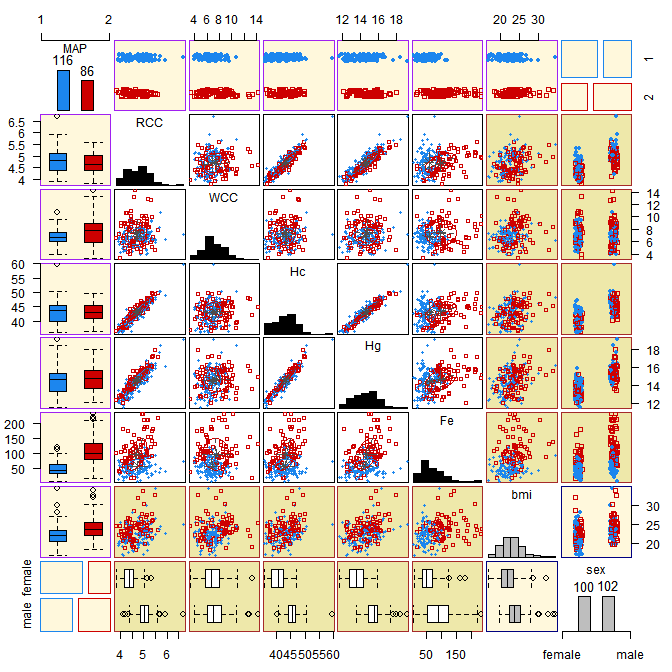Plot the BIC of the visited models.

plot(mod, what="criterion")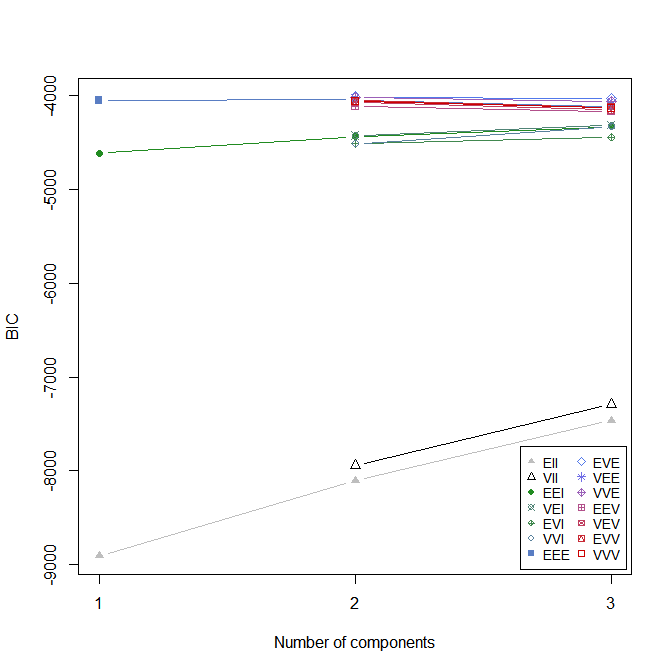For the optimal model, plot the gating network and the log-likelihood vs. EM iterations.

plot(mod, what="gating")
plot(mod, what="loglik")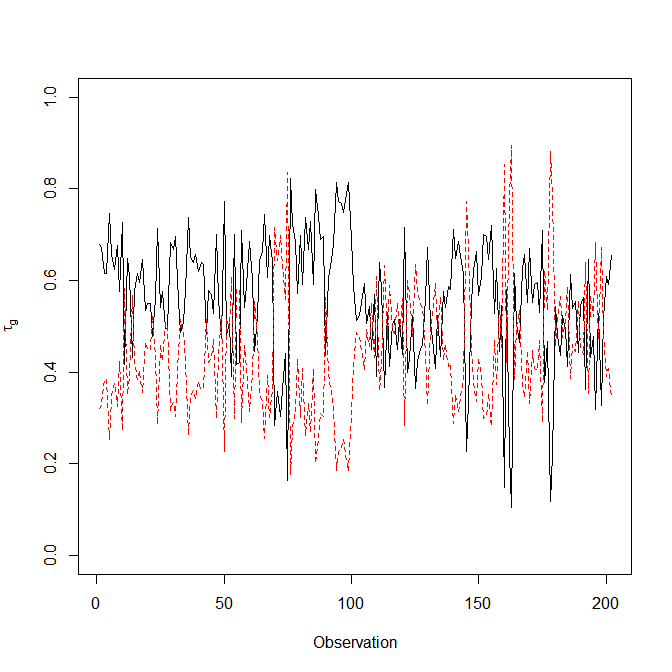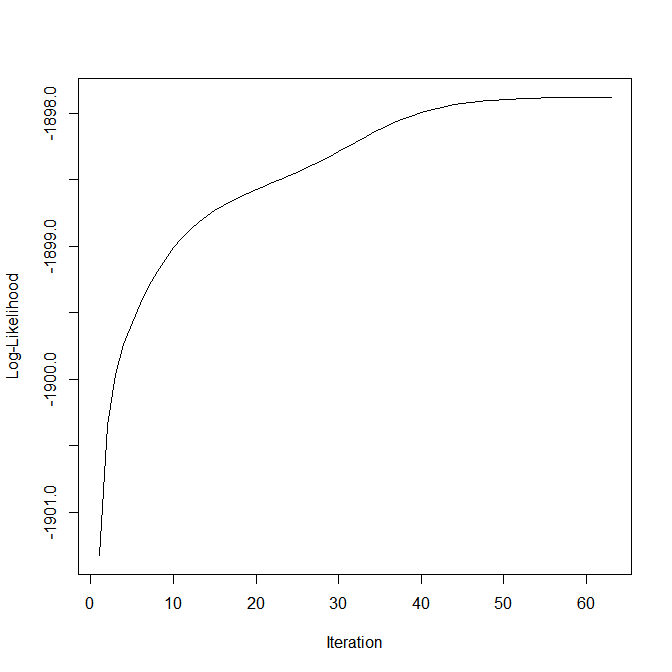Produce further visualisations with the aid of the lattice library.

require("lattice")
z <- factor(mod$classification, labels=paste0("Cluster", seq_len(mod$G)))
splom(~ hema | sex, groups=z)
splom(~ hema | z, groups=sex)